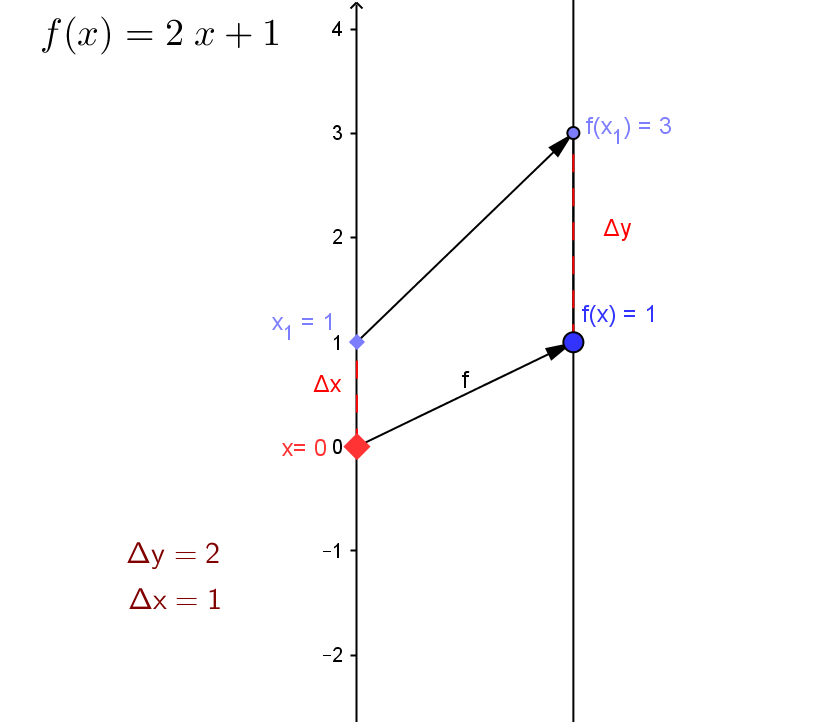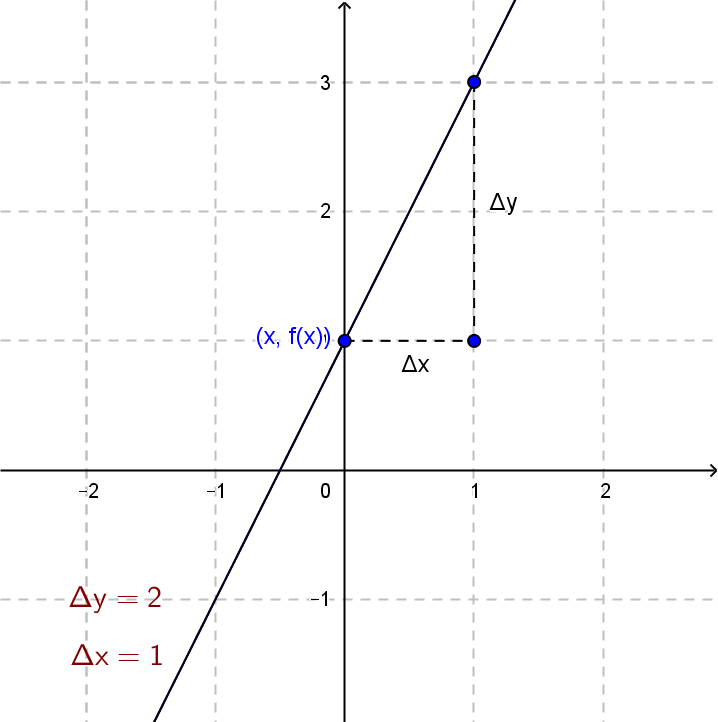Example 1: \$m  = 2; b = 1: f (x) = 2x + 1\$

Draw a mapping diagram and graph with a visualization for \$f\$ on the graph and a mapping diagram for \$x=0\$ and \$x_1=1\$ or use the diagram created with GeoGebra to explore further.

 Mapping Diagram for \$ f (x) = 2x + 1\$ \$x=0,x_1=1\$ Graph for \$ f (x) = 2x + 1\$On the GeoGebra figure you can move either \$x\$ or \$x_1\$ on the mapping diagram.
On the mapping diagram, given any two distinct points / numbers, \$x\$ and \$x_1\$, in the domain, the two corresponding arrows will not intersect between the two axes.
On the graph, if \$x<x_1\$, then the point \$(x,f(x))\$ will be lower and to the left of \$(x_1,f(x_1))\$.
Both these visual features are indicative of a increasing function.